Volumetric Analysis Lab Report for determining the concentration of an unknown substance in an aqueous solution.

Volumetric Analysis

Objectives

• To leam and practice quantitative techniques of Volumetric Analysis
• To standardize a sodium hydroxide solution
• To determine the molar concentration of a strong acid

# Introduction

Volumetric analysis is a common technique used to determine the concentration of an unknown substance in an aqueous solution. In volumetric analysis, volumetric glassware (e.g. burets, pipets, volumetric flask) is used to deliver a known quantity ofone substance, which reacts with an unknown amount of another substance, both as aqueous solutions.

Volumetric analysis is generally conducted by a titration procedure. Titration involves dispensing a solution of known concentration (the ‘titrant”) from a buret to a solution of unknown concentration (the “analyte”) in an Erlenmeyer flask. The titration procedure is particularly well-suited to acid-base neutralization reactions and allows the detennination ofconcentrations of unknown acids or bases with a high degree of accuracy and precision.

An acid-base neutralization reaction occurs when stoichiometric amounts of aqueous acid and base are combined. This is known as the equivalence point (or stoichiometricpoint), where moles of acid (H+) are equivalent to moles of base (OH-). The net ionic equation for a neutralization reaction between a strong acid and strong base is shown below:

1–1+ (an + OH- (an -+ H20 (0 (Eqn. 1)

The equivalence point ofa neutralization reaction is often detected using a visual indicator. Indicators are organic compounds which undergo dramatic color changes in well-defined pH rmges. An indicator is selected so that the equivalence point of the titration coincides (at approx. the same pH) with the point at which the indicator changes color, which is called the endpoint ofthe indicator. A common indicator for neutralization reactions is phenolphthalein, which is colorless in acidic solution but pink in basic solution.

For the acid-base titrdion studied in this experiment, the titrant is a standardized solution ofthe strong base, sodium (NaOH), and the analyte is a strong acid. As OH- is added to the acidic analyte, the acid is neutralized and the equivalence point is reached. At this point the solution is still colorless. As soon as a slight excess of OH- is added to the analyte, the excess base binds to the Indicator to produce a faint but pennanent color change. Consequently, the endpoint is achieved when the color change persists for at least 30 – 60 seconds. If done correctly, the volume of base used to reach the endpoint will be very similar to the volume needed to reach the equivalence point; hence, the endpoint can substitute for the equivalence point. A darker color change indicates that too much base has been added beyond the equivalence point, and will result in inaccurate calculations.

By carefully measuring the amount oftitrant used, one can determine the moles of analyte present, and hence its concentration. To determine the concentration of an acid, a standard solution of base (one whose concentration is known precisely), is prepared and used to react with the analyte. Concentration of both solutions is usually expressed in units of Molarity (M).

mol of solute

Concentration (M) =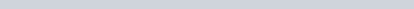of solution (Eqn. 2)volume (L)

NaOH is the titrant used in this experiment but it is hygroscopic. Being hygroscopic, solid NaOH absorbs water vapor from the aünosphere which results in an approximate concentration ofNaOH solution. Consequently, the NaOH

Fa1118 1

12

titrant will need to be standardized to determine its precise concentration by using a pnmary standard A primary standard is typically a reagent with the following properties:

d

• high punty
• high molar mass to reduce massing errors
• non-hygroscopic so that it remarns unchanged in air and stable during storage
• reacts in a direct, well-defined way

In Part A, the NaOH titrant will be standardized using a pnmary standard to determine the precise concentration of the NaOH solution. This information will then be used to calculate the molar concentration (molarity) of the strong acid in Part B. NOTE: The titrant for both Part A and Part B will be NaOH and will remain in the buret.

Part A Part B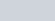Buret clampBuret

Titrant

NaOH

AnalyteBuret

Titrant

NaOH

Analyte

Buret stand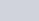Figure I : Titration setup for Parts A and B. Part A has KHP as analyte and Part B has unknown concentration ofHCl as analyte.

## Part A. Standardization of Sodium Hydroxide (NaOH) Solution

Since solid sodium hydroxide is highly hygroscopic its mass value is inaccurate. Therefore a solution ofNaOH of approximate molarity must be prepared and standardized with a primary standard. Potassium hydrogen phthalate (KHC8H404) is used as a primary standard in this experiment. Abbreviated as “KHP”, it is a large molecule with a molar mass of 204.23 g/mol, where “P” stands for the phthalate ion, (C8H404)2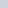A standard solution ofKHP is prepared by measuring a precise mass ofKHP and dissolving it in deionized water.

Molar mass can then be used to calculate the moles of KHPmass of KHP (g) mol ofKHP =molar mass of KHP (204.23g/mol) (Eqn. 3)

The pnmary standard solution ofKHP is then titrated with the NaOH solution until the end point when the indicator color changes from colorless to very light pink KHP is a monoprotic acid (one ionizable proton, H+) and reacts with NaOH in a l: I stoichiometric ratio according to the following balanced equation:

HKC8H404 (an + NaOH (an -+ NaKC8H404 (aq) + H20 (1) (Eqn. 4)

2t the end point (assuming end point = equivalence point), the moles ofNaOH that react with the KHP can be

determined:

1 mol NaOH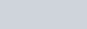mol NaOH = mol KHP (from Eqn. 3) X(Eqn. 5)

1 mol

The molar concentration of die sodium hydroxide solution can then be determined as:

mol of NaOHMolarity of NaOH =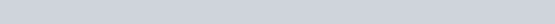(Eqn. 6) volume of NaOH dispensed (L)

Once the molar concentration ofNaOH is calculated, the NaOH solution is standardized and is now called a secondary standard solution.

 In order to standardize an approximate concentration of 0.15M NaOH, students need to determine the mass of KHP needc If approx. 16 mL ofNaOH solution is preferred to be used during each titration in Part A (a convenient amount), then the mass ofKHP needed can be calculated by the following method:O.016LNaOH x 0.15 mol NaOHx 1 molKHP x 204.23 gKHP 0.4902 g KHP 1 L solution 1 mol NaOH 1 mol KI-IPA student will need approximately 0.5 g of KHP to use about 16 mL of 0.15MNaOH for each titration.

Part B. Determination of the Molar Concentration (Molarity) of an Acid SolutionThe standardized NaOH solution is now ready to be used to titrate a measured volume of the strong acid to the end point. By knowing the volume ofNaOH dispensed through the buret and the molarity ofNaOH (from Part A), the moles ofNaOH are determined:

mol NaOH = Molarity of NaOH (from Eqn. 6) x volume dispensed (L) (Eqn. 7)

For a monoprotic acid HA (e.g., HCI), there is l: I stoichiometric reaction with NaOH given as:

HA (aq) + NaOH (an –4 NaA (aq) + H20 (0 (Eqn. 8)

Consequently, at the end point (assuming end point = equivalence point), the moles of acid that react with the NaOH can be determined:

1 mol acid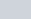mol of acid = mol NaOH (from Eqn. 7) x (Eqn. 9) 1 mol NaOHFinally, the molarity of the acid solution can be determined:mol of acid (from Eqn. 9)

M of acid =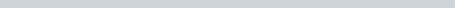(Eqn. 10)

volume of acid (L)3

• avoid skin contact, wear disposable gloves.Volumetric

Analysis

NaOH

and

HCI

are

corrosive

All

masses

measured

in

this

experiment must be recorded to 0.0001g (4 decimal places).

• All volumes measured from the buret must be recorded to two (2) decimal places.Part A. Standardize the NaOH Solution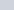Materials: Buret stand, buret clamp, titration funnel, 50-mL buret, 125 mL Erlenmeyer flasks (3), 50- or 100-mL measuring cylinder, 250 mL waste beaker, white sheet of paper.

1. Prepare a dilute NaOH solution of approximately 0.15 M concentration:
• Add approximately 400 mL of deionized water to a 500-mL polyethylene bottle. Add approximately 4 mL of concentrated (—18 M) NaOH solution, then add additional deionized water to dilute to 500 mL (dark ring at top of bottle). Cap the bottle tightly, mix well by inverting the boule repeatedly (do not shake). This dilute NaOH solution will be used for all titrations.
2. Prepare the buret: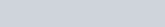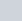Rinse a 50-mL buret with 5-mL portions of deionized water (3 times) making certain that the solution wets the entire inner surface. Rinse the buret with the dilute NaOH solution prepared in Step I (2 times) in the same manner as the water. Discard the rinses m a waste container, or as directed by your Instructor.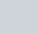Using a clean titration funnel, fill the buret with the dilute NaOH solution prepared in Step l. Do not fill theburet by pouring solution above your head — position the buret so that the solution and buret are at chest level. Place the buret in the buret clamp, with a 250mL waste beaker below the buret. Check for any leakage around the stopcock, and eliminate air bubbles in the tip of the buret by allowing a brief stream of solution to flow into the waste beaker. Record the Initial Volume to two decimal places by viewing the volume at the bottom of the meniscus.

1. Prepare the primary standard KHP solutions:

Prepare all three trials at the same time using three labeled Erlenmeyer flasks. Obtain three weigh boats and label the bottoms as l, 2 and 3.

• Tare weigh boat # l. Remove the boat from the balance to add approximately 0.5 gran-I ofthe solid acid KHP to the boat; record the mass to 4 decimal places. (Do not add KHP to the weigh boat while it is on the balance.) Repeat the process with fre other two weigh boats.
• Transfer each sample ofKHPto a clean, labeled 125-mL Erlenmeyer flask. Measure and pour approximately 50 mL of deionized water into each Erlenmeyer flask using the measuring cylinder to dissolve each sample.
• Add 2 drops of phenolphthalein indicator to each Erlenmeyer flask.

Check with instructor before going on to the next stage.

1.Titrate each KHP solution with NaOH solution:
• Place the Erlenmeyer flask with the KHP solution under the buret. Place a white sheet of paper under theflask• Dispense the NaOH solution slowly into the KHP solution. Swirl the flask with each addition.
• As you add NaOH, notice the pink color of the indicator as the NaOH enters the Erlenmeyer flask. As the pink color in the Erlenmeyer begins to persist, dccreasc the rate of addition, adding NaOH dropwise. The endpoint of ffe titration occurs upon addition of the drop that tums the solution the lightest pink color that persists for at least 60 seconds.
• Record the Final Volume of the buret to 2 decimal places.

Refill the buret (if necessary), record the new Initial Volume ofNaOH, and repeat the titration with the additional two trials.

Disposal and Cleanup

• Keep NaOH solution for use in Part B.
• Clean balance pan with brush and dispose of any spilled KHP in solid waste container. Close balance doors.
• Dispose of neutralized solutions in the Erlenmeyer flasks in the sink with plenty of running tap water. Rinse the three Erlenmeyer flasks with deionized water and have ready for Part B.

Part B: Determine the Molar Concentration of an Acid Solution

Equipment: Continue with the setup from Part A using the three rinsed Erlenmeyer flasks1. Prepare the buret:

• Reuse the buret setup from Part A. Fill the buretwith standardized NaOH solution. Record the Initial Volume reading to 2 decimal places.

1. Prepare the acid solution of unknown concentration: Prepare all three trials at the same time using the three Erlenmeyer flasks• Using a volumetric pipet, add 25.00 mL ofthe acid solution to each of three 125-mL Erlenmeyer flasks.
• Add 2 drops ofphenolphthalein indicator to each flask.

Check with instructor before going on to ffe next stage.

1. Titrate each acid sample with NaOH Solution:
• Dispense the NaOH solution slowly into the acid solution. Swirl the flask with each addition. As the pink color in the Edenmeyer begins to persist, decrease the rate of addition, adding NaOH dropwise. The end point of the titration occurs upon addition ofthe drop that tums the solution the lightest pink color that persists for at least 60 seconds.
• Record the Final Volume of the buretto 2 decimal places.

Refill the buret (if necessary), record the new Initial Volume ofNaOH, and repeat the titration with the additional two trials.

Calculations: Complete the calculations on the Report Sheet.

Disposal and Cleanup

• Dispose of all neutralized solutions in the sink with plenty of runmng tap water.
• NaOHin the polyethylene bottle should remain tightly capped, or dispose of as directed by instructor.
• Rinseffe buret, volumetric pipet, titration funnel, beakers and flasks with copious amounts of tap water, then rinse mice with deionized water. Retum all glassware and equipment to original storage locations.

5volumetric Analysis

## Prelaboratory Assignment

Date: Name: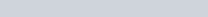Name of Partner:

l . a) What are some of the Important properties of a primary standard?

1. What is the pnmary standard used in this experiment? Give its name and formula.
2. Why can we not use NaOH as a primary standard?
3. In this experiment,
1. What is the name of titrant?1. What is the name of indicator?
2. What is the color change at the endpoint? to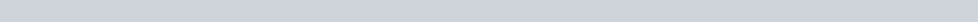1. Differentiate between an equivalence point and an endpoint in an acid-base titration.
2. What is a monoprotic acid? What is the stoichiometric ratio of its reaction with NaOH solution? Provide an example.
3. Calculate fre mass ofKHP needed to neutralize 18 mL of 0.15 M NaOH solution.
4. A scientist conducted the volumetric analysis using both Parts A and B and obtained the following data:

0.4985g KHP (molar mass of 204.23 g/mol ) was titrated to endpoint with 15.05mL ofNaOH as in Part A. Then as in

Part B, 25.0mL of unknown concentration ofHCl was titrated to the endpoint with 21.50mL ofNaOH. What is the

concentration of HCI?

Mass ofKHP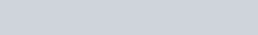Moles of KHP•Moles of NaOH at equivalence point: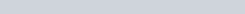Volume ofNaOH used to neutralize KHP• Molarity ofNaOH in the buret:Volume ofNaOH used to neutralize HCI: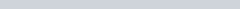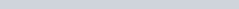Moles ofNaOH used to neutralize HCI: Moles ofHCl: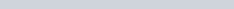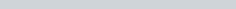Volume ofHCl used for titration: Molarity of HCI: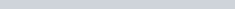7Volumetric

Analysis

## Report Sheet

Date: Name: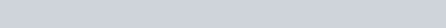Partner(s) Name:

Part A: Standardization of NaOH Solution

Data Table:

 Trial 1 Trial 2 Trial 3 Tared mass of KHP (g) 0. 2 P)’ 0211-(2 Molar mass of KHP (g/mol) 204.23 204.23 204.23 Moles of KHP (mol) – f, Y 49310 –Buret Reading: Initial Volume ofNaOH (mL) 0, 03642 c 0102+9 C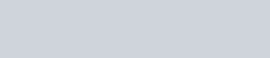Instructor’s signature Buret Reading: Final Volume ofNaOH (mL) Volume ofNaOH dispensed (mL) Molarity ofNaOH (mol/L)(For accurate work, the three molarities should be within ±5 %.)04 Avera e Molari of NaOH (mol/L 0/04 v (M

Show Entire Sample Calculation for Trial 1 here:

9

Part B: Determine the Molar Concentration of an Acid Solution

1. Unknown Acid Sample code:
2. Balanced equation for neutralization of acid with NaOH:
3.Data Table:
 Trial 1 Trial 2 Trial 3 Volume of acid solution (mL) 25.0 25.0 25.0 Buret Reading: Initial Volume ofNaOH (mL) 45.34 ml q C 4 5 gv.nl Instructor’s signatureBuret Reading: Final Volume ofNaOH (mL)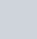95m)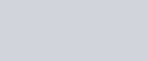25101 m/ Volume ofNaOH dispensed (mL) 2 1 455 Vh)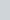Average molar concentration ofNaOH (mol/L), Part A Mols ofNaOH dispensed (mol) Molarity ofacid solution (mol/L)(For accurate work, the three molarities should be within ±5 %.)Avera e Molari of acid solution mol/L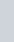Show Entire Sample Calculation for Trial 1 here:

10

Post-lab Questions Date:Name: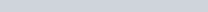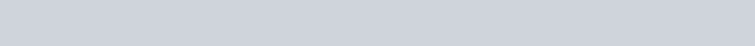Of Partner:

l. If the potassium hydrogen phthalate is not completely dry, how would it affect the reported molar concentration

(molarity) ofthe NaOH solution in Part A? Explain1.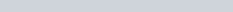In Part A, the mass ofKHC8H404 is measured to the nearest 0.0001 g. However, the volume of water used to dissolve the KHP does not need to be precise. Explain why the amount of water added to KHP has no effect on the experimentalresults.volumetric

Analysis

1. Identifrtwo possible sources of experimental error in this experiment, and describe the effect of each error in the determination of the molar concentration (molarity) of the acid solution in Part B.

If